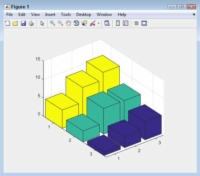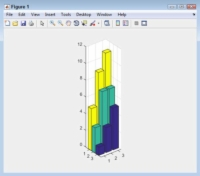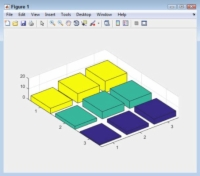How to Modify the Plot Box Aspect Ratio to Change the Appearance of Your MATLAB Plot - dummies

# How to Modify the Plot Box Aspect Ratio to Change the Appearance of Your MATLAB Plot

MATLAB allows you to modify the plot box aspect ratio. Instead of modifying the data, the plot box aspect ratio modifies the plot box — the element that holds the plot in its entirety — as a whole. The appearance of the data still changes, but in a different way than before. The following steps get you started with this example:

## 1Type YSource = [1, 3, 5; 3, 7, 9; 5, 7, 11]; and press Enter.

MATLAB creates a data source for you.

## 2Type Bar1 = bar3(YSource); and press Enter.

You see a 3D bar chart appear.## 3Type rotate(Bar1, [0, 0, 1], 270); and press Enter.

The bar chart rotates so that you can see the individual bars easier.

## 4Type pbaspect() and press Enter.

As before, you get three values: x-, y-, and z-axis. However, the numbers differ from before because now you’re working with the plot box aspect ratio and not the data aspect ratio. Here are typical output values at this point:

```ans =
2.8000 4.0000 2.4721```## 5Type pbaspect([1.5, 1.5, 7]); and press Enter.

The differences between the data points seem immense.

Notice how changing the plot box aspect ratio affects both the plot box and the data so that the plot box no longer is able to change settings, such as the spacing between bars. What this means in particular is that you don’t have to worry about bars ending up outside the plot area and not being displayed. The bars and the plot box are now locked together.## 6Type pbaspect([4, 5, 1]); and press Enter.

The data points now seem closer together, even though nothing has changed in the data.

## 7Type pbaspect(‘auto’); and press Enter.

The plot aspect returns to its original state.